# Connecting Mathematica to a C++ class library

## Examples from finance

(C) Weber & Partner, 2000

#### Introduction

Connecting Mathematica to a C function via MathLink is described in detail in the Mathematica book. With the examples provided for MathLink and the utility program mprep it is straightforward to implement the call to a valuation routine for a financial instrument written in C from Mathematica. But in the documentation for MathLink the connection of Mathematica to a  C++ class libraries is not discussed. MathLink is compatible with C++ compilers but does itself not follow the object oriented paradigm. In our understanding using an object oriented approach is what distinguishes C++ code from C code.
In this notebook we will show that connecting Mathematica to a class library is technically no more complicated than calling a C function. In fact the generic structure of Mathematica and MathLink enables you to take full advantage of the benefits of a class library thereby significantly reducing the amount of needed implementation work and allowing to write compact but powerful Mathematica programs.

### Theory

A class library is usually built up using inheritance to establish a polymorphic type hierarchy, where specific subclasses implement the behaviour and semantics defined by more generic superclasses. C++ class libraries for financial instruments or valuation routines do not deviate from that pattern. As an example take the pricing or valuation function: Each concrete type of instrument implements the abstract method defined higher in the hierarchy using the valuation model appropriate for its kind (see for example Fowler (1999), Rogers (1997), Infinity (1998),  Algorithmics (1997)).

What is gained from this polymorphism besides other benefits is the ability to treat collections of different objects in a generic way. A situation where this is helpful is for example the valuation of a portfolio which consists of diverse instruments like an exotic option book. This philosophy of generic programming  matches extremly well with Mathematica since its programming model is based on the same principles. To make the match in principle it is only necessary to establish a mapping between the objects of the class library and objects in Mathematica that makes the objects' generic interface available through MathLink. In the context of financial instruments this interface may consist of methods to create an financial instrument, let it load its parameters from a database, let it price itself etc. To make this kind of functionality available in Mathematica only very few lines of C code are necessary. Additionally some infrastructure is needed that handles aspects like the life cycle of objects, type conversions, exceptions etc. This infrastructure tends to be generic. It can be programmed once and reused whenever necessary.

### Example

In this sections an example is given which was written to demonstrate the advocated approach.

•  This loads a Mathematica package which wraps the MathLink interface to the pricing functionality provided from a tiny C++-class library.

``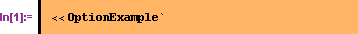``

While loading the package the MathLink program is started and a new context OptionExample is created. Wrapping the interface allows to further simplify the Mathematica code through the use of options and Mathematica's advanced pattern matching. It is also the place to introduce error checking, help and other functionality which would need a lot of programming either in the MathLink interface or in the class library.

#### Creating and pricing options

Two kinds of options are modelled in the class library. One is a simple call option, the other is a Down-and-Out call option.

•  The function CreateCallOption

``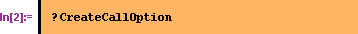``
`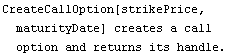`

creates a simple call option and returns a handle to the instantiated object. As arguments the function accepts the strike price of the option and its maturity date specified as list of the form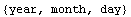.

•  The function CreateDownAndOutOption

````
`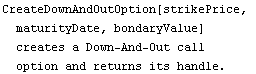`

creates a Down-and-Out option. The function  expects as additional argument the barrier for its underlying's share price. If the share price touches or falls below the barrier, the option is knocked out.

•  To calculate the price of an option the function PriceOption is provided.

``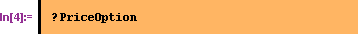``
`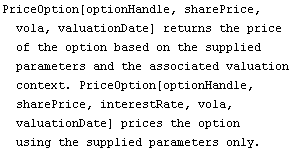`

There exist two overloaded versions of this function. The version used here expects the current share price, the interest rate, the share's volatility and the valuation date as arguments, in other words all the parameters necessary for the analytic valuation.

These functions suffice to create an option and calculate its value. As first example a call option with a strike of 70 (units of currency) and maturity at 2. April 2001 is priced. It is valuated assuming an underlying stock price of 68, an interest rate of 5% and a volatility of 0.2. The valuation date is the 10. October 2000.

``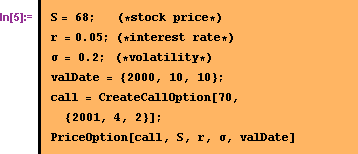``
``

In the same way a Down-and-Out option can be valuated. However, it is necessary to specify the knock-out boundary as additional parameter. Here 60 is chosen:

``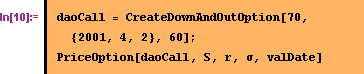``
`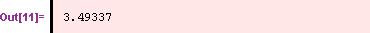`

As can be seen above, the pricing function is applied to the different kinds of options in the same way. Therefore options of either kind can be put into a single portfolio and valuated together:

````
``

#### Valuation using market scenarios

The value of a financial instrument usually depends upon additional extrinsic parameters that characterize the state of the instrument's environment, i. e. the market. Therefore class libraries often introduce independent objects that comprise the relevant parameters and thus represent a market scenario. This separation is essential when studying the value of the financial instrument for a multitude of different market scenarios as is done e. g. in risk management.

•   For the sake of simplicity the market scenarios in this example are made up only of interest rates. Two different types of market scenarios (or valuation contexts, as they are called here) are provided: The first kind assumes a constant interest rate for all periods and is created through a call to the function CreateSimpleContext.

````
`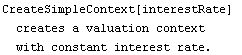`

•  The second kind supports arbitrary relations between interest rates and terms. In the implementation another feature of Mathematica is used: External programs can send an arbitrary expression back to the Mathematica kernel and retrieve the result of the evaluation. This callback mechanism allows to extend class libraries with Mathematica programs in a seamless way. To create this kind of valuation context the function CreateCallbackContext is called.

``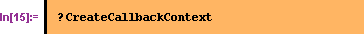``
``

The argument interestCurve stands for a symbol defined in Mathematica that should be bound to a function which returns an interest rate for a supplied period.

•  The option has to know which valuation context it should use when it is asked to calculate its price. To associate the option with a valuation  context the function AssociateContext is used.

````
`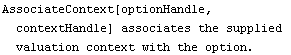`

As arguments the handles of the option and the valuation context are expected.

•  To calculate the price of an option using a market scenario the second form of function PriceOption is needed.

````
``

This version of the pricing function is called with only four parameters, the missing interest rate is supplied by the associated valuation context. To demonstrate this a valuation context with a constant interest rate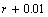is created and used to valuate the simple call option from above.

``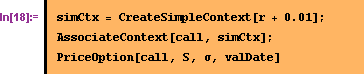``
``

Compare this result with the price calculated with an interest rateusing the first form of  PriceOption:

``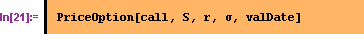``
`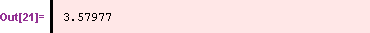`

The next example shows how to use the valuation context that employs Mathematica's callback mechanism. A callback context is set up that refers to symbol rate. This implies that the following contract for symbol rate is guaranteed: The pattern rate[t_Real] must be bound to an expression that returns a number. As long as this condition is satisfied the specific expression is arbitrary. Here rate[t_Real] just returns the value of the global variable aRate. Every time the class library accesses this valuation context Mathematica is asked to evaluate the variable. The class library does not notice this special behaviour since it accesses the valuation context through the interface of the parent class.

``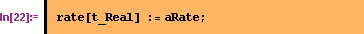``

Now the callback context can be created and associated with the call option:

``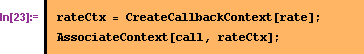``

The next expression changes the value of aRate from 0.01 to 0.2 in increments of 0.01. At each step the option is priced. The list of calculated prices is then plotted.

``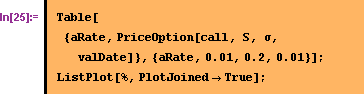``A more common use of this mechanism would be to define an interest rate curve from sampling points using Mathematica's interpolation functions.

``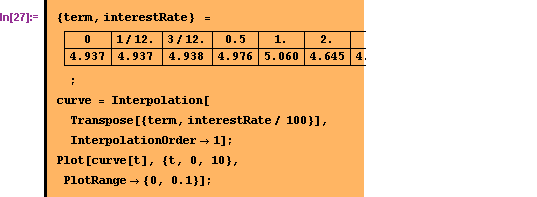``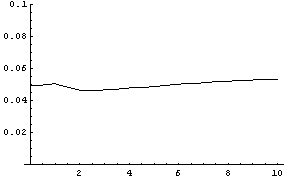To use this interest rate curve as market scenario a valuation context is created that refers to the symbol curve. It is then associated with the call option.

``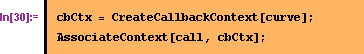``

Now the call option is priced with the interest rate curve:

``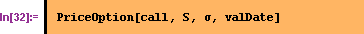``
`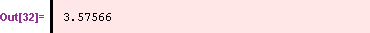`

As was mentioned the different objects of the class library interact with each other on the basis of their generic interfaces, so any kind of option can be combined with any kind of valuation context, e. g. a Down-and-Out option with an callback context:

``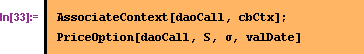``
``

#### Valuation of multiple options

For the last example another function is introduced that returns information about the object associated with a particular handle. This kind of functionality becomes essential when dealing with groups of objects.

•  After an object was created and associated with a handle its properties can be retrieved with function

``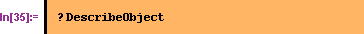``
``

The function returns the state of the associated object as a list of the form {"property1"->value1, ..., "propertyN"->valueN}.

A portfolio consisting of multiple options with different maturities and strike prices based on a common underlying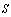is set up.  Instead of the simplistic way used here in practice more elaborate schemes taking advantage of Mathematica's advanced statistical functions would be employed.

``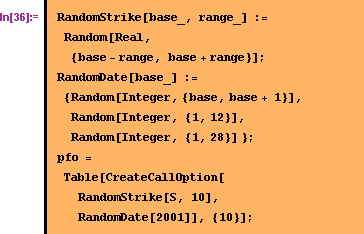``

The options in the portfolio are associated with the interest rate curve that was defined above:

``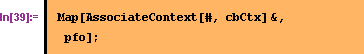``

Only a few lines are necessary to calculate the option prices and display the results in a table:

``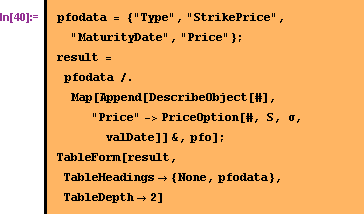``
 Type StrikePrice MaturityDate Price SimpleOption 73.07900576918271`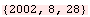7.923363225840184` SimpleOption 59.92533410932385`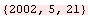14.394781467689853` SimpleOption 66.93074044947377`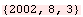10.697937019819648` SimpleOption 76.34335220350627`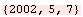5.627267055890627` SimpleOption 59.885952194655275`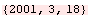9.937397395901499` SimpleOption 70.74079223875859`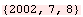8.523419437754761` SimpleOption 71.50507233539419`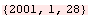1.9625992501486351` SimpleOption 68.29538142621567`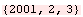3.440331636712898` SimpleOption 67.14794768899696`3.5355804944732796` SimpleOption 62.31756582345244`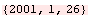7.246926952580601`

The combined portfolio value is given as

``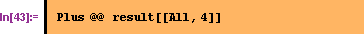``
`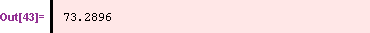`

A second portfolio consisting of Down-And-Out options is set up using the properties of the options in the first portfolio. The strike price of an option is also used as its knock-out boundary value.

``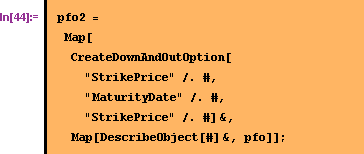``

The portfolio is valuated with the same interest rate curve as above. Note that the valuation works the same regardless whether the portfolio consists of plain vanilla or Down-And-Out options.

``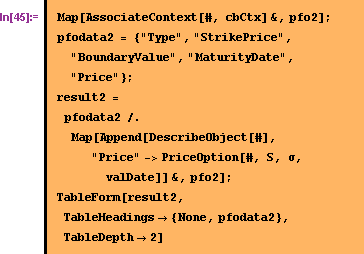``
 Type StrikePrice BoundaryValue MaturityDate Price DownAndOutOption 73.07900576918271` 73.07900576918271`0.` DownAndOutOption 59.92533410932385` 59.92533410932385`10.841421075071157` DownAndOutOption 66.93074044947377` 66.93074044947377`1.6291212598934663` DownAndOutOption 76.34335220350627` 76.34335220350627`0.` DownAndOutOption 59.885952194655275` 59.885952194655275`9.206037557813636` DownAndOutOption 70.74079223875859` 70.74079223875859`0.` DownAndOutOption 71.50507233539419` 71.50507233539419`0.` DownAndOutOption 68.29538142621567` 68.29538142621567`0.` DownAndOutOption 67.14794768899696` 67.14794768899696`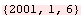1.0085199358096872` DownAndOutOption 62.31756582345244` 62.31756582345244`6.392770004743523`

The combined value of the Down-And-Out options in this portfolio is:

``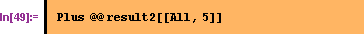``
`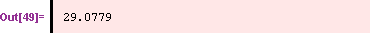`

This illustrates how useful Mathematica's notion of representing data in lists and its ability to apply operations on each element as well as the entire lists gets when analysing sets of possibly different objects. Here the ideal match between the generic programming models exhibited by Mathematica and object oriented class libraries becomes obvious.
This concludes the example, the external program can be terminated.

````
`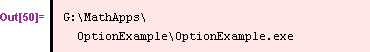`

### Practical experience

The approach described above was used to make the functionality of the C++ class library from Infinity (Fin++) available under Mathematica. This combination of Mathematica and a financial domain C++ class library proved valuable in several projects for a client in the banking industry, e. g. the validation of the internal market risk model through a risk estimate based on Monte Carlo simulation. From the experience gained in these projects it can be concluded that once the basic framework for the object mapping between the class library and Mathematica is established the extension of the mapping to comprise more classes is a comparatively minor task. The framework itsself defines the structure of the mapping and thereby a process to make the library's objects available in Mathematica. The source code provided in the example demonstrates only some aspects found in such a framework, a more complete implementation would contain e. g. classes for the automatic conversion of data types, generic ways to instantiate classes (class factories), send messages to them, etc. Through a pattern based approach a reliable and extensible framework design can be accomplished. On the other side with the callback mechanism demonstrated above it is possible to seamlessly incorporate Mathematica code for rapid prototyping into the class library without breaking the library's semantics.

### References

Algorithmics Inc. (1997), Risk++ User's Guide.
Fowler, Martin